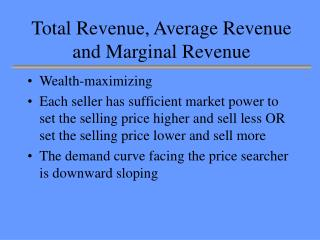DownloadDownload PresentationTotal Revenue, Average Revenue and Marginal Revenue

# Total Revenue, Average Revenue and Marginal Revenue

Download Presentation## Total Revenue, Average Revenue and Marginal Revenue

- - - - - - - - - - - - - - - - - - - - - - - - - - - E N D - - - - - - - - - - - - - - - - - - - - - - - - - - -
##### Presentation Transcript

1. Total Revenue, Average Revenue and Marginal Revenue • Wealth-maximizing • Each seller has sufficient market power to set the selling price higher and sell less OR set the selling price lower and sell more • The demand curve facing the price searcher is downward sloping

2. Total Revenue, Average Revenue and Marginal Revenue • Total revenue ( TR ) is the total amount of money(or some other good) that a firm receives from the sale of its goods. It the firm practices single pricing rather than price discrimination, TR = total expenditure of the consumer = P x Q

3. Total Revenue, Average Revenue and Marginal Revenue • Average revenue ( AR ) is the total amount of money(or some other good) that a firm receives from the sale divided by the number of units of goods sold. • AR = TR/Q, since TR=P x Q, then AR = P for single pricing practice • And since MUV = DD = P, then • MUV = DD = P = AR

4. Total Revenue, Average Revenue and Marginal Revenue • Marginal revenue ( MR ) is the change in total revenue resulting from selling an extra unit of goods. • MR = TR/Q, where TR = change in TR due to change in Q, Q = change in Q

5. To find T R from the M R curve • For a certain known quantity transacted, the area under the MR and above the horizontal axis is the T R . (I.e. the sum of the Marginal Revenues of all units of goods.) • The slope of the TR curve is MR. Why? • And, MR is always smaller Price for single pricing arrangement (I.e. MR < P) Why? (Hint MR<AR, AR=P for single pricing)

6. For a certain known quantity transacted, the area under the MR and above the horizontal axis is the T R . (I.e. the sum of the Marginal Revenues of all units of goods, I.e. area 0ACQ) Also, TR = AR x Q, I.e. area 0PBQ Price A B P C AR MR 0 Q Quantity

7. \$ Slope at point E = MR TR E slope =AR 0 Quantity The slope of the TR curve is MR The relations between TR, AR and MR

8. Total Revenue, Average Revenue and Marginal Revenue • The slope of Marginal revenue ( MR ) is twice the slope of AR. • Why? (See next slide) • (The relations between TR, AR and MR can also be applied to TUV, AUV and MUV)

9. P, AR, MR = AR curve MR curve AR Total Revenue TR MUV = DD = P = AR Q Quantity

10. P, AR, MR = AR curve MR curve AR Total Revenue TR TR MUV = DD = P = AR Q Quantity

11. P, AR, MR = AR curve AR MR curve MUV = DD = P = AR Q Quantity

12. Total Revenue, Average Revenue and Marginal Revenue • The areas of the 2 triangles must be the same for total revenue should be the same. • The two triangles must be the same only if the MR cuts the midpoint of the perpendicular line drawn from the DD to the vertical axis. • Hence, the slope of Marginal revenue ( MR ) is twice the slope of AR.

13. The relationship between AR and MR • The slope of MR is twice the slope of AR • MR curve is not the demand curve (the relationship between price and quantity). • However, if the price searcher practises price discrimination or All-or-Nothing Pricing Arrangement, then All-or-nothing pricing = • All-or-nothing DD = AUV , which is > MUV , and also downward-sloping

14. Revision on different pricing arrangement • Single Pricing Arrangement • with Consumer Surplus = TUV - TEV • MUV = DD = AR = P [>MR] {<AUV} • All-or-Nothing Pricing Arrangement • All consumer surplus will be extracted, so that TUV = TEV • hence, All-or-Nothing DD curve = All-or-nothing pricing = AUV {>MUV}

15. REVISION Single Pricing Arrangement All-or-nothing Pricing Arrangement DEMAND AUV AUV = AR P P DEMAND MUV = MR MUV = AR MR Q Q TUV= TEV TUV= TEV + CS

16. A Price -Searcher = Price-Searcher’s Market MR cuts the midpoint of the perpendicular line drawn from the AR to the vertical axis. Price AR MR Quantity